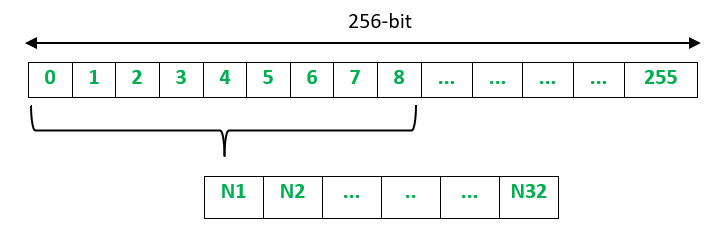Skip to content
Related Articles
Winternitz One Time Signature Scheme
• Last Updated : 02 Jun, 2020

Winternitz One Time Signature (WOTS) is a quantum resistant digital signature scheme that uses relatively small key and signature sizes. As it is a one-time signature scheme, it can only be used to securely sign one message.

Suppose Alice wants to digitally sign her message to Bob, the process can be explained in 3 steps: Key Generation, Signature Generation and Signature Verification.

Key Generation :

1. Alice needs to create a key pair – private and public key.
2. To create the private key, a random number generator is used to generate 32 256-bit random numbers. The private key is known only to Alice.
3. To create the public key, each of the 32 numbers is hashed 256 times to obtain another set of 32 256-bit numbers. The public key is shared with everyone.

Signature Generation :

1. Alice hashes the message using SHA 256 which produces a 256-bit digest. This digest is split up into 32 8-bit values (N1, N2, …, N32).2. Alice hashes each of the 8-bit value 256-N times, where N is the value of the 8-bit value. For example, if N1 is the 8-bit value is 10001000 = 136, then N1 would be hashed 256-136 = 120 times. After doing this for each of the 8-bit values, the digital signature is generated.

Signature Verification :

1. Bob hashes the message using SHA-256 to produce the digest of 32 8-bit values (N1, N2, …, N32).
2. Bob then hash the signature value by the number of times given by the message hash value (N1, N2, …, N32).
3. Bob compares the result with Alice’s public key. If they are a match, the signature is valid.

Attention reader! Don’t stop learning now. Get hold of all the important CS Theory concepts for SDE interviews with the CS Theory Course at a student-friendly price and become industry ready.

My Personal Notes arrow_drop_up